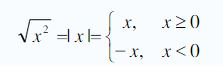Ontario Tech acknowledges the lands and people of the Mississaugas of Scugog Island First Nation.

We are thankful to be welcome on these lands in friendship. The lands we are situated on are covered by the Williams Treaties and are the traditional territory of the Mississaugas, a branch of the greater Anishinaabeg Nation, including Algonquin, Ojibway, Odawa and Pottawatomi. These lands remain home to many Indigenous nations and peoples.

We acknowledge this land out of respect for the Indigenous nations who have cared for Turtle Island, also called North America, from before the arrival of settler peoples until this day. Most importantly, we acknowledge that the history of these lands has been tainted by poor treatment and a lack of friendship with the First Nations who call them home.

This history is something we are all affected by because we are all treaty people in Canada. We all have a shared history to reflect on, and each of us is affected by this history in different ways. Our past defines our present, but if we move forward as friends and allies, then it does not have to define our future.

# Square Root

Let’s take a look at the concept of taking the square root of a number.

## Understanding the Square Root

To square a number, just multiply that number by itself. For example, 32 = 9. A square root works in the opposite way. For instance, if you square 3, you get 9, and if you "take the square root of 9", you get 3 (i.e. 32 = 9 so √9 = 3). In general, a square root of a number is a value that can be multiplied by itself to give the original number. The “√” symbol is called the "radical" symbol. The expression "√9" is either read as "root nine", "radical nine", or "the square root of nine".

Example:

Find the square root of the number 25.

Solution:   √25 = 5 since (5)2 = 25

The largest misconception about the use of a square root is this: √9 = ± 3. This is not correct. Square roots are always positive, so the correct value is √9 = 3. Note that the value of the simplified radical is positive and this is the only value of the square root and this positive result is called the “principle” root. While either of +3 and –3 might have been squared to get 9, "the square root of nine" is defined to be only the positive option, +3. If we want the -3 then we do the following:

-√9 = - (√9 ) = - (3) = -3.

Notice that the parentheses are used to make the point on just how the minus sign is appearing. In general the middle two steps are omitted. So, if we want the negative value we have to actually put in the minus sign.

This misconception arises because we are sometimes asked to solve things like x2 = 9. When you solve the equation     x2 = 9,  you are trying to find all possible values that might have been squared to get 9. Here, the answer is x2= ± 3 and often this will be solved by “taking the square root” of both sides. Here is the proper solution for this problem:

x2 = 9

x = ±√9

x = ± 3

Note that the ± shows up in the second step before the square root is evaluated. It does not show up as part of taking the square root.

For all real numbers x, we have the following piecewise relationship:Example:

Solve the equation 2x2 + 8 = 40

Solution:

2x2 + 8 = 40

2x2 = 32

x2 = 16

x =  ±√16

x = ± 4

Avoiding Common Math Mistakes with Square Roots: